# JP3869814B2 - Orthorectification processing method for satellite images - Google Patents

## Info

Publication number
JP3869814B2
JP3869814B2 JP2003069813A JP2003069813A JP3869814B2 JP 3869814 B2 JP3869814 B2 JP 3869814B2 JP 2003069813 A JP2003069813 A JP 2003069813A JP 2003069813 A JP2003069813 A JP 2003069813A JP 3869814 B2 JP3869814 B2 JP 3869814B2
Authority
JP
Japan
Prior art keywords
image
block
pixel position
obtained
reference
Prior art date
Legal status (The legal status is an assumption and is not a legal conclusion. Google has not performed a legal analysis and makes no representation as to the accuracy of the status listed.)
Active
Application number
JP2003069813A
Other languages
Japanese (ja)
Other versions
JP2003323611A (en
JP2003323611A6 (en
Inventor

Original Assignee
ヒタチソフトウエア グローバル テクノロジー リミテッド

Priority date (The priority date is an assumption and is not a legal conclusion. Google has not performed a legal analysis and makes no representation as to the accuracy of the date listed.)
Filing date
Publication date
Priority to US10/128,586 priority Critical patent/US6810153B2/en
Priority to US10/128586 priority
Application filed by ヒタチソフトウエア グローバル テクノロジー リミテッド, 日立ソフトウエアエンジニアリング株式会社 filed Critical ヒタチソフトウエア グローバル テクノロジー リミテッド
Publication of JP2003323611A6 publication Critical patent/JP2003323611A6/en
Publication of JP2003323611A publication Critical patent/JP2003323611A/en
Application granted granted Critical
Publication of JP3869814B2 publication Critical patent/JP3869814B2/en
Active legal-status Critical Current
Anticipated expiration legal-status Critical

• 238000003672 processing method Methods 0 title claims description 5
• 230000000875 corresponding Effects 0 claims description 28
• 238000000034 methods Methods 0 claims description 9
• 238000004364 calculation methods Methods 0 description 42
• 241001441724 Tetraodontidae Species 0 description 5
• 230000000694 effects Effects 0 description 2

## Images

•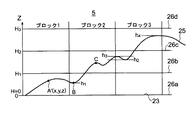•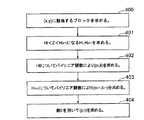•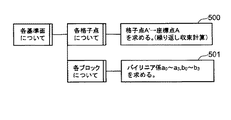•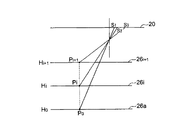•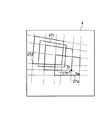•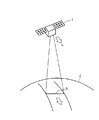•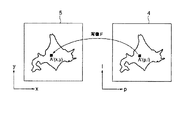•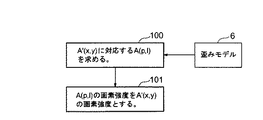•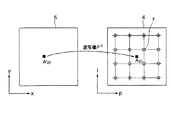•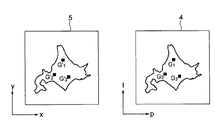••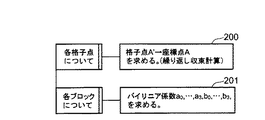•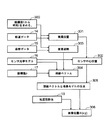••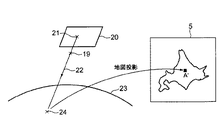•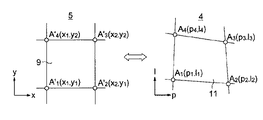•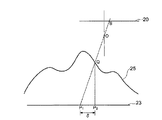## Classifications

• GPHYSICS
• G06COMPUTING; CALCULATING; COUNTING
• G06TIMAGE DATA PROCESSING OR GENERATION, IN GENERAL
• G06T3/00Geometric image transformation in the plane of the image
• G06T3/0068Geometric image transformation in the plane of the image for image registration, e.g. elastic snapping
• G06T3/0081Geometric image transformation in the plane of the image for image registration, e.g. elastic snapping by elastic snapping
• GPHYSICS
• G06COMPUTING; CALCULATING; COUNTING
• G06TIMAGE DATA PROCESSING OR GENERATION, IN GENERAL
• G06T5/00Image enhancement or restoration
• G06T5/006Geometric correction

## Description


BACKGROUND OF THE INVENTION
The present invention relates to an image processing method for correcting distortion of a ground image taken from a satellite, and more particularly to an ortho correction processing method for correcting distortion caused by undulations on the ground surface.

[Prior art]
First, an image (satellite image) taken from a satellite and the principle of correction will be described.

FIG. 6 is an explanatory diagram of image capturing by a satellite, where 1 is a satellite, 2 is the ground surface, and 3 is a ground observation line.

In the figure, the satellite 1 images the ground surface 2 by detecting electromagnetic waves from the ground surface 2 with a sensor (not shown) mounted downward. When such a sensor is a line sensor such as a CCD that is generally used as a satellite sensor, the sensor is arranged so that the arrangement direction of one row of cells (sensor elements) constituting the sensor is orthogonal to the traveling direction L of the satellite 1. The range on the ground surface 2 that is arranged and observed simultaneously by such a sensor is the ground surface observation line 3. The ground observation line 3 moves in the traveling direction L together with the satellite 1, and along with this movement, detection data of each cell is taken in at a predetermined sampling period. The detection data is taken in at the same time for all cells. The data of the entire ground observation line 3 corresponds to one line of the satellite image. Therefore, data corresponding to one line of the photographed image is acquired by one data acquisition (one sampling). In this way, the surface 2 is continuously observed with a certain width (Swath) by the surface observation line 3 as the satellite 1 advances in the orbit.

FIG. 7 is a diagram showing the principle of correction of the satellite image obtained in this way. 4 is an observation image and 5 is a correction image.

In the same figure, the above-mentioned image captured by the satellite is sent to the ground as an observation image 4. The observed image 4 is distorted in the shape of the image due to factors such as fluctuations in the orbit and attitude of the satellite, distortion of the optical system of the sensor, and the shape of the earth surface. For this reason, in order to use the captured image by the satellite, it is necessary to correct the distortion of the observed image 4 and convert it into a corrected image 5 according to a predetermined map projection method. Here, such correction is performed by mapping from the pixel A (p, l) of the observed image 4 to the corresponding pixel A ′ (x, y) of the corrected image 5, and this is called mapping F. .

The observation image 4 is represented by a pl coordinate system in which the sensor cell array direction on the satellite 1 (that is, the longitudinal direction of the ground observation line 3) is p-coordinate and the traveling direction L of the satellite 1 is l-coordinate. Further, the corrected image 5 is represented by an xy coordinate system in which the direction corresponding to the p coordinate of the observed image 4 is the x coordinate and the direction corresponding to the l coordinate of the observed image 4 is the y coordinate.

FIG. 8 is a flowchart showing the correction processing of the observed image 4.

In the corrected image 5 shown in FIG. 7, the pixels are arranged and set at equal intervals. On the other hand, the observed image 4 corresponds to each pixel of the corrected image 5 due to the distortion described above. Pixel positions are not arranged at regular intervals. In the correction processing shown in FIG. 8, for each pixel position in the corrected image 5, a pixel at the pixel position in the observation image 4 corresponding to the pixel position is found and positioned at the corresponding pixel position in the corrected image 5. It is.

In FIG. 8, such correction includes distortion modeling 100 and resampling 101. In the distortion modeling 100, for each pixel position in the corrected image 5, a pixel position in the observation image 4 corresponding to the pixel position is obtained. Referring to FIG. 7, the position of the pixel A (p, l) in the observed image 4 corresponding to the position of the pixel A ′ (x, y) in the corrected image 5 is obtained using a distortion model 6 created in advance. Is. For this purpose, it is necessary to obtain an inverse map F-1 of the map F.

Here, the distortion model 6 is predicted from the orbital and attitude fluctuations of the satellite at the time of capturing the observation image 4, the distortion of the optical system of the sensor, or the like obtained from the observation result of the satellite. It is.

In the resampling 101, when the position of the pixel A (p, l) of the observation image 4 corresponding to the position of the pixel A ′ (x, y) in the corrected image 5 is obtained by the distortion modeling 100, the pixel A The intensity of the pixel at the position (p, l) is obtained and used as the intensity of the pixel at the pixel A ′ (x, y) of the corrected image 5.

FIG. 9 is an explanatory diagram of the resampling 101 in FIG. 8 and will be described using the observed image 4 and the corrected image 5 shown in FIG. Reference numeral 7 denotes a center position of the pixel in the observation image 4 (hereinafter referred to as a pixel position).

In this figure, the position of the pixel A (p, l) of the observed image 4 corresponding to the position of the pixel A ′ (x, y) of the corrected image 5 is obtained by the distortion modeling 100 described in FIG. The position of the pixel A (p, l) generally does not coincide with the pixel position 7 of the observation data by the sensor, as shown in the figure. Therefore, the intensity of the observation data at the position of the pixel A (p, l) needs to be obtained by interpolation from the pixel intensity at the pixel position 7 actually obtained around the position of the pixel A (p, l). . As an interpolation method for this purpose, interpolation equations such as Nearest Neighbor, Bilinear, and Cubic Convolution are generally used.

Conventionally, as a first correction method of satellite observation image data based on the above principle, an ortho correction method is known (for example, see Non-Patent Document 1).

This represents the distortion modeling 100 of FIG.

[Expression 1]
This equation is called the Rational polynomial method, and for each captured image, the coefficients of the x, y, and z polynomials will be described below by using a ground control point (GCP). Thus, the position of the observed image 4 with respect to each pixel position of the corrected image 5 is obtained by Equation 1 using the obtained coefficient.

FIG. 10 is an explanatory diagram of a first correction method using such a ground reference point.

In the figure, in the observed image 4, a plurality of ground control points G i (i = 1, 2,...), for example, three ground control points G 1 , G 2 , G Three Is selected. Such ground reference points can be clearly identified in shape and position in the observation image 4, and the position coordinates in the correction image 5 corresponding to the positions of these ground reference points are read from a map or surveyed. Is exactly what you get. In the corrected image 5, these ground control points G 1 , G 2 , G Three The position corresponding to 1 ', G 2 ', G Three Shown as'.

In this way, a plurality of ground control points G in the observation image 4 i (P i , L i ) And the corresponding position G in the corrected image 5 i '(X i , Y i , Z i ) And these ground control points G i (P i , L i ) Pixel coordinate value and position G i '(x i , Y i , Z i ), The unknown coefficient of the polynomial expressed by Equation 1 can be obtained by the least square method, whereby the inverse mapping F -1 Is possible. Note that the degree of the polynomial is appropriately set according to the number of ground control points GCP and the situation of distortion.

According to this first method, if there is altitude data DEM (Digital Elevation Model) and ground reference point in the observation target area, distortion of the satellite can be obtained without satellite orbit data, attitude data, or satellite sensor optical model. There is an advantage that modeling and correction processing using the same are possible.

As a conventional second correction method for satellite observation image data, the following method is also known as a non-ortho correction, that is, a method that does not consider undulations due to the elevation of the ground surface. This is a method based on a geometric model using satellite orbit data, attitude data, and an optical model of a satellite sensor. This method is characterized in that the corrected image is divided into blocks by equidistant grids, and a distortion model expression is obtained for each block.

FIG. 11 is a diagram for explaining block division by a lattice in the second correction method.

In the figure, in the corrected image 5, grids 8 are set at equal intervals in the vertical and horizontal directions, and the corrected image 5 is divided into a plurality of blocks 9 by the grid 8. The pixel positions in the corrected image 5 are divided into blocks by such blocks 9. Then, the position of the observation image 4 corresponding to each lattice point 10 where these lattices 8 intersect is inversely mapped F. -1 The lattice 13 having the obtained positions obtained as lattice points 12 is set, and the observation image 4 is also divided into blocks 11 by the lattice 13. As an example, the lattice point A in the observed image 4 is the inverse map F of the lattice point A ′ in the corrected image 5. -1 It is shown that the lattice points A ′ and A correspond to each other.

The block 11 of the observed image 4 corresponds to one of the blocks 9 of the corrected image 5, and the blocks 11 and 9 of the observed image 4 and the corrected image 5 correspond to each other one to one. The blocks 9 and 11 are set so that the distortion is approximately uniform among them, and the distortion may be different between adjacent blocks. However, the inverse mapping F so that the distortion continues at the boundary between adjacent blocks. -1 Is set.

Here, reverse mapping F -1 As follows, the bilinear function expressed by the following equation 2 is used.

[Expression 2]
Here, Equation 2 is composed of 0th-order and 1st-order terms of x and y, and a term of xy, so that distortion continues at the boundary between adjacent blocks. X 2 , Y 2 Including these higher-order terms causes distortion discontinuities (steps) at the boundary between adjacent blocks. To eliminate this, add many higher-order terms and add the whole screen. Must be calculated as one block, and the calculation becomes very complicated and time consuming. Here, the images 4 and 5 are divided into blocks having such a size that the distortion can be regarded as uniform, and a bilinear function is obtained for each block, whereby the inverse mapping F -1 Therefore, the inverse map F is obtained by a simple expression such as Equation 2. -1 Can be done. In this case, as described above, by adding the xy term, distortion is continued at the boundary between adjacent blocks.

FIG. 12 shows the coefficient a in the above bilinear function. 0 , A 1 , A 2 , A Three , B 0 , B 1 , B 2 , B Three It is a flowchart which shows the calculation method of.

In this figure, this calculation includes a lattice point calculation 200 and a coefficient calculation 201.

Referring to FIG. 11 as an example, the lattice point calculation 200 is to obtain the coordinate position A (p, l) in the observed image 4 for each lattice point A ′ (x, y) in the corrected image 5. This includes map F -1 However, this is generally obtained directly without the ground reference point, and thus is obtained by using a recursive convergence operation of the geometric calculation of the map F.

FIG. 13 is a flowchart showing the geometric calculation of the map F, and FIG. 14 is a diagram showing an arrangement of a part of pixel positions of the observed image 4. FIG. 15 is a diagram for explaining the geometric calculation of the map F. The map F is to obtain a lattice point A ′ (x, y) with respect to the coordinate value A (p, l). The trajectory data 14, the posture data 15, the sensor optical model 16, and the pixel position data 17 in FIG. 13 correspond to the distortion model 6 in FIG.

In FIG. 14, four pixel positions A 1 (p 1 , L 1 ), A 2 (p 2 , L 1 ), A Three (p 2 , L 2 ), A Four (p 1 , L 2 ). First, pixel position A 1 (p 1 , L 1 ) As an example, the calculation shown in FIG. 13 will be described.

13 and 15, the pixel position A 1 (P 1 , L 1 ) Coordinate value l 1 To its pixel position A 1 Is obtained (step 300). The coordinate value l from the orbit data 14 of the satellite 1 and the obtained photographing time t 1 The position (satellite position) in the inertial space of the satellite 1 at the time of photographing is obtained (step 301), and the coordinate value l is obtained from this satellite position and the optical model 16 of the sensor in the satellite 1. 1 The position (sensor optical center) 19 in the inertial space of the optical center of the sensor at the time of photographing is obtained (step 302).

Further, the attitude (satellite attitude) of the satellite 1 in the inertial space at the imaging time t is obtained from the imaging time t obtained in step 300 and the attitude data 15 of the satellite 1 (step 303). Optical model 16 and pixel position data 17 (here, pixel position A 1 (P 1 , L 1 ) Coordinate value p 1 ) From this coordinate value p on the sensor surface 20 1 Pixel position A passing through the position 21 of the sensor element (ie, cell) corresponding to 1 (P 1 , L 1 ) Is determined in the inertial space (step 304). Then, an intersection 24 between the line-of-sight vector 22 extending from the sensor optical center 19 obtained in step 302 and the ground model 23 is obtained (step 305). This intersection 24 is the pixel position A of the observed image 4 1 (P 1 , L 1 ) Represents the position indicated by the latitude and longitude on the ground surface model 23, and the pixel position A in the corrected image 5 is calculated by this and a predetermined map projection method 18. 1 (P 1 , L 1 ) To obtain a coordinate position A ′ (x, y). As the ground model 23, a spheroid is usually used.

By the way, the pixel position A obtained in this way. 1 (P 1 , L 1 The coordinate position A ′ (x, y) corresponding to) does not coincide with the grid point 10 (FIG. 11) set in the corrected image 5. Therefore, the pixel position A in FIG. 1 (P 1 , L 1 ) Adjacent pixel position, eg, pixel position A 2 (P 2 , L 1 ) And the same calculation is performed for this, and the pixel position A in the corrected image 5 is calculated. 2 (P 2 , L 1 ) To obtain a coordinate position A ′ (x, y). If this still does not coincide with the grid point 10 set in the corrected image 5, another pixel position is selected again, the same calculation is performed, and the calculation is repeated, and the calculation result becomes the corrected image. The pixel position A (p, p) of the observation image 4 corresponding to the grid point A ′ (x, y) of the corrected image 5 is calculated by, for example, calculation about 4 to 5 times. l) is approximately obtained.

Then, such calculation is performed for each grid point 10 of the corrected image 5, and the pixel position A (p, l) of the observation image 4 corresponding to each grid point 10 of the corrected image 5 is obtained. The above is the lattice point calculation 200 in FIG.

In the coefficient calculation 201 in FIG. 12, the coefficient a of the bilinear function expressed by the above equation 2 for each block 9 of the corrected image 5 shown in FIG. 0 , A 1 , A 2 , A Three , B 1 , B 2 , B Three Is what you want. That is, a bilinear function is obtained for each block 9.

For example, as shown in FIG. 16, if the block 11 in the observed image 4 corresponds to the block 9 in the corrected image 5, the pixels in the block 11 are inversely mapped F into the block 9. -1 It is this coefficient calculation 201 that obtains a bilinear function for this purpose. For this purpose, using the coordinate values of the lattice points at the four corners of these blocks 9, 11, a 0 , A 1 , A 2 , A Three , B 1 , B 2 , B Three Ask for.

Here, four corner grid points A in the block 9 1 '(x 1 , Y 1 ), A 2 '(x 2 , Y 1 ), A Three '(x 2 , Y 2 ), A Four '(x 1 , Y 2 ) Is a grid point (pixel position) A at the four corners of the block 11. 1 (P 1 , L 1 ), A 2 (P 2 , L 2 ), A Three (P Three , L Three ), A Four (P Four , L Four ), And therefore, the coordinate pair ((x 1 , Y 1 ), (P 1 , L 1 )), ((X 2 , Y 1 ), (P 2 , L 2 )), ((X 2 , Y 2 ), (P Three , L Three )), ((X 1 , Y 2 ), (P Four , L Four )), The simultaneous equations shown in the following equation 3, that is,
[Equation 3]
To solve for the desired coefficient a 0 , A 1 , A 2 , A Three , B 1 , B 2 , B Three Is obtained. By substituting these coefficients thus obtained into Equation 2, a bilinear function for these blocks 9 and 11 is obtained, and this indicates the pixel position in the block 11 relative to the pixel position in the block 9. Needless to say, when the pixel position in the block 9 does not coincide with the pixel position in the block 11 by the bilinear function, the interpolation processing as described in FIG. 9 is performed.

Then, by performing the coefficient calculation 201 (FIG. 12) as described above for each block 9 of the corrected image 5, a bilinear function for each block 9 is obtained, and the inverse from the observed image 4 to the corrected image 5 is obtained. Map F -1 Is possible.

This second method includes:
(1) Since it is a bilinear model for each block, there is no divergence of errors caused by the least square method as in the first method.
(2) The distortion model is continuous at the block boundary.
(3) Since Equation 3 is used, the amount of calculation for calculating the position coordinates of each point of the corrected image is smaller than that of the polynomial in Equation 1.
(4) Modeling is possible without ground control points.
(5) If there is a ground reference point, the error contained in the orbital data and attitude data of the satellite is estimated and corrected to improve the modeling accuracy.

[Non-Patent Document 1]
Demystification of IKONOS by Thierry Toutin and Philip Cheng Earth Observation Magazine vol.9, No.7, pp.17-21,2000

[Problems to be solved by the invention]
By the way, in the first correction method,
(1) Ground control points are essential for modeling, and cannot be applied when ground control points cannot be obtained in areas where maps are not available.
(2) The accuracy of modeling depends on the number and arrangement of ground control points, and depending on the number and arrangement, the estimation accuracy of the coefficients of the polynomial may deteriorate, and in particular, errors other than the ground control points may diverge. ,
(3) For each corrected image, the calculation of the above equation 1 is required, and the amount of calculation for correction processing increases.
There was a problem.

In addition, since the second correction method is approximated by a spheroid model in which the ground surface does not undulate, orthorectification considering the fallen distortion caused by the undulation of the ground in consideration of the elevation data DEM of the observation area Has a problem that it cannot be applied as it is.

Here, with reference to FIG. 17, the collapse distortion due to the undulation of the ground will be described.

In the figure, according to the second correction method, there is actually a undulating ground surface 25 with respect to the ground model 23 of the spheroid. Therefore, when the observation image 4 is obtained as described above by photographing the satellite 10, when looking at the cell S on the sensor surface 20, the cell S is obtained from the sensor optical center 19 and the line-of-sight vector obtained as described above. 22 and intersection point P with the ground surface model 23. 1 Is the pixel position of the corrected image, but in reality, the intersection P between the vertical line passing through the intersection Q with the undulating ground surface 25 and the surface model 23 2 Is a correct pixel position of the corrected image. These intersection points P 1 And intersection P 2 The misalignment on the corrected image corresponding to the misalignment is referred to as collapse distortion δ.

The object of the present invention is to eliminate such problems, without requiring a ground reference point, without divergence of the error in the entire image, without being excessively complicated, and to prevent collapse distortion caused by the undulation of the ground surface. An object of the present invention is to provide a method for orthorectifying satellite images that can be corrected.

[Means for Solving the Problems]
In order to achieve the above object, the present invention sets a plurality of reference planes having different altitudes relative to a reference plane of zero altitude above the sea level of the reference spheroid earth model, and blocks a corrected image with an equally spaced grid. The model equation of distortion by a bilinear function is obtained for each block and for each reference surface of the plurality of elevations.

Further, the present invention uses the model formulas of the two reference planes of the upper and lower elevations sandwiching the elevation value of the pixel position in the corresponding block for each pixel position of the corrected image, and the observation corresponding to each elevation value. The pixel position of the image is calculated, and the pixel position of the observation image is obtained by linearly interpolating these two pixel positions of the observation image based on the value of the altitude data.

DETAILED DESCRIPTION OF THE INVENTION
Hereinafter, embodiments of the present invention will be described with reference to the drawings.

FIG. 1 is an explanatory diagram showing an embodiment of a method for orthorectifying a satellite image according to the present invention, and reference numerals 26a, 26b, 26c, and 26d denote reference planes.

In the figure, the ground surface 25 is shown in cross section, and a plurality of reference planes 26a, 26b, 26c, and 26d having different elevations are set on the corrected image 5. Here, the reference plane 26a is zero above the sea level of the ground model 23 of the reference spheroid (H O = 0) and the reference surface 26b is at an altitude H 1 Reference plane 26c is at an altitude H 2 The reference plane 26d is at an altitude H Three The reference plane of H 0 <H 1 <H 2 <H Three It is. Elevation H 1 , H 2 , H Three Are appropriately set as desired.

In FIG. 1, blocks 1, 2, and 3 are shown. Then, a bilinear function on each reference plane is obtained for each block, and by using this, a pixel position in the observation image with respect to the pixel position is obtained for each reference plane. The correspondence between the pixel position on the ground surface 25 and the pixel position on the observation image 4 is obtained using the correspondence relation between the pixel position on the reference plane and the pixel position on the observation image 4 thus obtained. Thus, distortion caused by the undulation of the ground surface 25 is eliminated.

Hereinafter, such processing will be described.

FIG. 2 is a flowchart showing the correction process of this embodiment, which corresponds to the distortion modeling process 100 in the correction process shown in FIG.

As shown in FIG. 1, a plurality of reference planes 26 a to 26 d are set in the corrected image 5, and the reference planes 26 a to 26 d are set so as to correspond to each other. The block is set by. The corrected image is divided into blocks by equally spaced grids.

Now, since the coordinate of the pixel of interest A ′ in the corrected image 4 is on the ground surface 25 where the image of interest A ′ undulates and has the elevation z given by the elevation data DEM, (x, y , Z).

Therefore, in FIG. 2, first, a corresponding block is obtained based on the coordinate value (x, y) of the pixel of interest A ′ (step 400). In the case of FIG. 1, block 1 is determined.

Next, based on the altitude z of the target pixel A ′, two reference planes that directly sandwich the position of the target pixel A ′ are obtained. This elevation value z is the elevation value H i (However, i = 0, 1, 2,...) Reference plane and elevation value H i + 1 Between the reference plane, i.e.,
[Expression 4]
The elevation value H i , H i + 1 Find the reference plane. In the case of FIG. 1, the elevation value H 0 Reference plane 26a and elevation H 1 The reference plane 26b is obtained (step 401). At point B of block 2, the altitude value H 0 Reference plane 26a and elevation H 1 The reference plane 26b is obtained, and at point C, the elevation value H 1 Reference plane 26b and elevation value H 2 The reference plane 26c is obtained.

And the calculated elevation value H i The coefficient a of the bilinear function expressed by the above formula 2 0 , A 1 , A 2 , A Three , B 0 , B 1 , B 2 , B Three Is calculated by the method described later, and the altitude value H is calculated using a bilinear function using these coefficients. i Pixel position A on the reference plane i '(x i , y i ) Coordinate value A in the observed image 5 i (P i , L i ) Is obtained (step 402). Similarly, the elevation value H i + 1 Pixel position A on the reference plane i + 1 '(x i + 1 , y i + 1 ) Coordinate value A in the observed image 4 i + 1 (p i + 1 , L i + 1 ) In the case of FIG. 0 Pixel position A on the reference plane 26a 0 '(x 0 , Y 0 , Z 0 ) Coordinate value A in the observed image 5 0 (P 0 , L 0 ) And elevation value H 1 Pixel position A on the reference plane 26b 1 '(x 1 , Y 1 , Z 1 ) Coordinate value A in the observed image 4 1 (P 1 , L 1 ) Is obtained (step 123).

Next, in the observed image 4, the coordinate value A i + 1 And coordinate value A i The coordinate value position A (p, l) with respect to the altitude z of the target pixel A ′ is
[Equation 5]
Obtained by linear interpolation (step 404).

FIG. 3 is a flowchart showing a bilinear function coefficient calculation method in steps 402 and 403 in FIG.

In the figure, the calculation described in FIG. 12 is performed for each reference plane having a different altitude. Here, the lattice point calculation 500 is the same as the lattice point calculation 200 in FIG. 12, and the coefficient calculation 501 is also the same as the coefficient calculation 201 in FIG. The geometric calculation of the map F in the lattice point calculation 500 is the same as that shown in FIG. However, in the calculation shown in FIG. i It is changed according to.

This will be explained with reference to FIG. 4. Elevation value H of the same block in the corrected image 5 0 , H i , H i + 1 Reference planes 26a, 26 i , 26 i + 1 , Position P on the reference plane 26a 0 On the other hand, the reference plane 26 i , 26 i + 1 Then, this position P 0 Position P directly above i , P i + 1 (Ie, position P 0 And the same latitude and longitude). Therefore, the position P on the reference plane 26a. 0 Cell on sensor surface 20 for S 1 Then, the reference plane 26 i Position P at i Cell at sensor surface 20 is cell S 2 And the reference plane 26 i + 1 Position P at i + 1 Cell at sensor surface 20 is cell S Three It is.

As described above, even at the same latitude and longitude, the cells differ according to the reference planes having different altitudes. Therefore, the process of making the cells different according to the reference planes, that is, the coordinate values in FIG. It is necessary to correct p and l.

FIG. 5 is a diagram showing mapping of lattice points in the observed image 4 with respect to a plurality of reference planes having different altitudes, and 27a, 27b, 27c, and 27d are blocks.

In the figure, the block 27a corresponds to the block 1 in the region with respect to the reference surface 26a in FIG. 1, the block 27b corresponds to the block 1 in the region with respect to the reference surface 26b, and the block 27c Similarly, block 1 corresponds to block 1 in the region relative to the reference surface 26c, and block 27d corresponds to block 1 in the region corresponding to the reference surface 26d. Thus, even in the same block of the corrected image 5, the blocks in the observed image 4 are different for each of the reference planes 26a, 26b, 26c, and 26d. This is because the coordinate value 17 in FIG. 13 is corrected according to the altitude in the lattice point calculation 500 in FIG.

When the lattice point position for each reference plane of the corrected image 5 and the pixel position in the observed image 4 are obtained by the lattice point calculation 500 in this way, the coefficient calculation 501 and the coefficient calculation 201 shown in FIG. Similarly, the coefficient a of the bilinear function expressed by the above equation 2 for each block on each reference plane. 0 , A 1 , A 2 , A Three , B 0 , B 1 , B 2 , B Three And a bilinear function for each block on each reference plane is obtained.

Therefore, for example, when the pixel position A in the observation image 4 with respect to the pixel position A ′ of the altitude value z of the block 1 in the corrected image 4 in FIG. 1 is obtained, this pixel position A is obtained by the obtained bilinear function. In the blocks 27a and 27b (FIG. 5) in the observed image 4 for the block 1 of the reference planes 26a and 26b directly sandwiching ', the pixel position A corresponding to the pixel position A' on the reference plane 26a a Pixel position A of observed image 4 with respect to ' a Is obtained from the block 27a, and the pixel position A corresponding to the pixel position A ′ on the reference plane 26b is obtained. b Pixel position A of observed image 4 with respect to ' b Are obtained from block 27b and these obtained pixel positions A a , A b Accordingly, by performing an interpolation process according to the altitude value z at the pixel position A ′, the pixel position A (p, l) in the observed image 4 with respect to the pixel position A ′ (x, y, z) in the corrected image 5. Will be obtained.

In addition, altitude H of reference plane i The selection of the following conditions:
(1) For each block, set a reference plane having a sufficient number of different elevations to cover the range between the minimum and maximum values of the elevation value data DEM in the block.
(2) The minimum reference elevation value exceeding the common part of the fluctuation range of the elevation value data DEM of each of the two adjacent blocks and the maximum below this common part so that the distortion model is continuous at the boundary between the adjacent blocks. All the reference plane elevation values that are combined with the reference elevation value of the same block must be set to a common value in these adjacent blocks.
Need to be considered.

Note that (2) will be described by taking blocks 2 and 3 in FIG. 1 as an example. The change in elevation value in block 2 is h. 1 ~ H Three And the change in altitude value in block 3 is h 2 ~ H Four It is. Where h 1 <H 2 <H Three <H Four , Altitude change range h 2 ~ H Three In this range, the altitude fluctuation range in block 2 overlaps with the altitude fluctuation range in block 3, which is the "range of fluctuation of elevation value data DEM in each of the two adjacent blocks" in blocks 2 and 3. Is the common part. In addition, the above-mentioned “minimum reference elevation value exceeding the common part” is the elevation value H 2 In addition, the “maximum reference altitude value below the common part” is the altitude value H 1 It is. And such elevation value H 1 , H 2 When a reference plane is set between them, the altitude value of the reference plane must be the same in block 2 and block 3.

Elevation H i Can be determined by the magnitude of fluctuation of the elevation value data DEM in the block and the off-needer angle of the block (the magnitude of the perspective angle measured from the point immediately below the satellite 10).

That is, it is only necessary that the sum of the approximation error of the bilinear model and the linear approximation error with respect to the altitude value of the fall-down amount be within the range of the allowable error after correction. Qualitatively, when the altitude fluctuation in the block is large and the squint angle is large, the amount of collapse and the linear approximation error thereof become large. i It is necessary to reduce the interval. The interval can be determined by estimating the approximation error in advance by model calculation or the like.

【The invention's effect】
As explained above, according to the present invention,
(1) Using the given elevation data DEM, it is possible to correct the collapse distortion caused by the undulations on the ground surface.
(2) Since it is a bilinear model for each block, there is no divergence of errors caused by finding the polynomial of the Rational polynomial method by the method of least squares.
(3) The distortion model is continuous at the block boundary.
(4) Modeling is possible without ground control points.
(5) If there is a ground reference point, use it to estimate the error contained in the satellite orbit data and attitude data, and correct it to improve the modeling accuracy and improve the correction accuracy. it can
The effect is obtained. Thereby, it is possible to provide a practical satellite image ortho-correction method in terms of both accuracy and calculation amount.
[Brief description of the drawings]
FIG. 1 is an explanatory diagram showing an embodiment of a method for ortho-correcting a satellite image according to the present invention.
FIG. 2 is a flowchart showing correction processing of the embodiment shown in FIG. 1;
FIG. 3 is a flowchart showing a method of calculating a bilinear function coefficient in steps 402 and 403 in FIG. 2;
4 is an explanatory diagram of lattice point calculation 500 in FIG. 3. FIG.
FIG. 5 is a diagram illustrating mapping of lattice points of an observation image with respect to a plurality of reference planes having different elevations.
FIG. 6 is an explanatory diagram of image capturing by a satellite.
FIG. 7 is a diagram illustrating the principle of satellite image correction.
FIG. 8 is a flowchart showing observation image correction processing;
9 is an explanatory diagram of resampling in FIG. 8. FIG.
FIG. 10 is an explanatory diagram of a first observation image correction method using a ground reference point.
FIG. 11 is a diagram for explaining lattice points and blocks in the second correction method of an observed image.
FIG. 12 is a flowchart illustrating a method of calculating a coefficient using a bilinear function.
13 is a flowchart showing geometric calculation of map F in lattice point calculation 200 in FIG.
FIG. 14 is a diagram illustrating an arrangement of a part of pixel positions of an observation image.
15 is a diagram for explaining the geometric calculation of the map F in FIG. 13;
FIG. 16 is a diagram for explaining the relationship between blocks in a corrected image and an observed image.
FIG. 17 is a diagram for explaining collapse distortion due to elevation.
[Explanation of symbols]
1 satellite
2 Ground
3 Ground observation lines
4 Observation images
5 Corrected image
6 Distortion model
7 pixel position
8 lattice
9 blocks
10 grid points
11 blocks
12 lattice points
13 lattice
14 Orbit data
15 Attitude data
16 Sensor optical model
17 Coordinate value
18 Map projection
19 Sensor optical center
20 Sensor surface
21 Sensor element position
22 eye vector
23 Surface model
24 intersection
25 Ground surface
26a-26d, 26 i , 26 i + 1 Reference plane
27a-27d block

## Claims (2)

1. Orthorectification of a satellite-captured image comprising a distortion modeling process for associating a pixel position of a correction image with a pixel position of an observation image obtained by a satellite, and a resampling process for interpolating the pixel intensity of the observation image according to the distortion modeling process In the processing method,
The distortion modeling process sets a plurality of reference planes having different elevations with respect to a reference plane of zero altitude above the sea level of the reference spheroid earth model. A first step of dividing into
In each of the blocks, a second step in which a sufficient number of different altitude reference values are set to cover a range of minimum and maximum altitude values at pixel positions in the block;
The elevation values of all the reference planes included between the minimum reference elevation value exceeding the common part of the range of fluctuation of the elevation values in the two adjacent blocks and the maximum reference elevation value below the common part. A third step whose value is common to these adjacent blocks;
Seeking the block corresponding to the pixel position for each pixel position of the corrected image is obtained to determine the two reference surfaces of the upper and lower sandwiching the altitude value of the pixel position in the block, obtained the in the block with upper and lower reference surfaces each on the obtained bilinear function due to distortion model formula, obtained under the upper sandwiching the altitude value of the pixel position in the block reference surface each two corresponding observed image to elevation values A pixel position is calculated, and the calculated two pixel positions are linearly interpolated according to the altitude value of the pixel position of the corrected image to obtain a pixel position of the observed image with respect to the pixel position in the corrected image . Steps and
Consists of
Orthorectification of the satellite observation image, wherein the processing of the second to fourth steps is repeated until all pixel positions in the observation image are obtained corresponding to the respective pixel positions in the correction image. Processing method.
2. In claim 1,
The interval between the elevations of the reference plane in each block is an approximation error of the bilinear model and a linear approximation error with respect to the elevation value of the fall-down amount according to the magnitude of fluctuation of the elevation value data in the block and the off-needia angle of the block. Of the satellite observation image is determined so that the sum of the two values falls within the allowable error range after the correction processing by repeating the processing in the second step to the fourth step. Method.
JP2003069813A 2002-03-20 2003-03-14 Orthorectification processing method for satellite images Active JP3869814B2 (en)

## Priority Applications (2)

Application Number Priority Date Filing Date Title
US10/128,586 US6810153B2 (en) 2002-03-20 2002-04-24 Method for orthocorrecting satellite-acquired image
US10/128586 2002-04-24

## Publications (3)

Publication Number Publication Date
JP2003323611A6 JP2003323611A6 (en) 2003-11-14
JP2003323611A JP2003323611A (en) 2003-11-14
JP3869814B2 true JP3869814B2 (en) 2007-01-17

# Family

## Family Applications (1)

Application Number Title Priority Date Filing Date
JP2003069813A Active JP3869814B2 (en) 2002-03-20 2003-03-14 Orthorectification processing method for satellite images

## Country Status (5)

US (1) US6810153B2 (en)
EP (1) EP1357516B1 (en)
JP (1) JP3869814B2 (en)
CN (1) CN100405398C (en)
DE (1) DE60330524D1 (en)

## Families Citing this family (26)

* Cited by examiner, † Cited by third party
Publication number Priority date Publication date Assignee Title
US7310440B1 (en) * 2001-07-13 2007-12-18 Bae Systems Information And Electronic Systems Integration Inc. Replacement sensor model for optimal image exploitation
KR100488685B1 (en) * 2002-08-22 2005-05-11 한국과학기술원 Image Processing Method for Automatic Image Registration and Correction
US7983446B2 (en) * 2003-07-18 2011-07-19 Lockheed Martin Corporation Method and apparatus for automatic object identification
US7324664B1 (en) * 2003-10-28 2008-01-29 Hewlett-Packard Development Company, L.P. Method of and system for determining angular orientation of an object
EP1899889A2 (en) * 2005-06-24 2008-03-19 Digitalglobe, Inc. Method and apparatus for determining a location associated with an image
JP2008506167A (en) * 2004-06-25 2008-02-28 デジタルグローブ インコーポレイテッドＤｉｇｉｔａｌｇｌｏｂｅ，Ｉｎｃ． Method and apparatus for determining a location associated with an image
US20060126959A1 (en) * 2004-12-13 2006-06-15 Digitalglobe, Inc. Method and apparatus for enhancing a digital image
EP1675299B1 (en) * 2004-12-23 2018-08-01 Hewlett-Packard Development Company, L.P. Authentication method using bilinear mappings
JP4523422B2 (en) * 2005-01-07 2010-08-11 三菱電機株式会社 Satellite image position correction device
US7660430B2 (en) * 2005-05-23 2010-02-09 Digitalglobe, Inc. Method and apparatus for determination of water pervious surfaces
TWI301707B (en) * 2006-02-16 2008-10-01 Univ Nat Central
JP4702122B2 (en) * 2006-03-15 2011-06-15 三菱電機株式会社 Orthorectifier for synthetic aperture radar images
KR100762891B1 (en) * 2006-03-23 2007-10-04 연세대학교 산학협력단 Method and apparatus of geometric correction of image using los vector adjustment model
US8155433B2 (en) 2008-07-10 2012-04-10 Goodrich Corporation Method of object location in airborne imagery using recursive quad space image processing
WO2010125041A1 (en) * 2009-04-27 2010-11-04 St-Ericsson (France) Sas Geometric method of transforming a two-dimensional image.
US8577186B1 (en) 2011-02-14 2013-11-05 DigitalOptics Corporation Europe Limited Forward interpolation approach using forward and backward mapping
US8675115B1 (en) 2011-02-14 2014-03-18 DigitalOptics Corporation Europe Limited Forward interpolation approach for constructing a second version of an image from a first version of the image
US8340462B1 (en) * 2011-02-14 2012-12-25 DigitalOptics Corporation Europe Limited Pixel mapping using a lookup table and linear approximation
CN102682481B (en) * 2012-05-28 2014-08-20 国家卫星气象中心 Method for determining geometrical characteristic information of earth under regional observation mode
US8942421B1 (en) * 2012-11-07 2015-01-27 Exelis, Inc. Geolocation of remotely sensed pixels by introspective landmarking
WO2014163069A1 (en) * 2013-04-01 2014-10-09 株式会社次世代技術研究所 Radar signal processing method and device
CN104463924B (en) * 2014-11-12 2017-02-15 南京师范大学 Digital elevation terrain model generation method based on scattered point elevation sample data
CN105717538B (en) * 2014-12-02 2018-02-02 中国石油天然气股份有限公司 Relief surface earthquake data offset reference plane conversion method and device
CN104977013A (en) * 2015-05-27 2015-10-14 无锡市崇安区科技创业服务中心 GPS navigation image treatment method
US10055885B2 (en) * 2015-09-16 2018-08-21 Raytheon Company Systems and methods for digital elevation map filters for three dimensional point clouds
KR101965965B1 (en) * 2017-09-05 2019-08-13 순천대학교 산학협력단 A method of automatic geometric correction of digital elevation model made from satellite images and provided rpc

## Family Cites Families (11)

* Cited by examiner, † Cited by third party
Publication number Priority date Publication date Assignee Title
DE3219032C3 (en) * 1982-05-19 1988-07-07 Messerschmitt Boelkow Blohm receptive stereo photogrammetric Metric and evaluation
JPH0526233B2 (en) * 1983-01-20 1993-04-15 Hitachi Ltd
JPH0563835B2 (en) * 1983-12-02 1993-09-13 Hitachi Ltd
FR2653918B1 (en) * 1989-10-31 1992-02-14 Europ Agence Spatiale Method for real-time rectification of images of geostationary meteorological satellites.
DE4419359A1 (en) * 1994-06-03 1995-12-07 Wolfram Dipl Ing Kirchner Procedure for the acquisition, evaluation, measurement and storage of geographic information
US5649032A (en) * 1994-11-14 1997-07-15 David Sarnoff Research Center, Inc. System for automatically aligning images to form a mosaic image
US6011505A (en) * 1996-07-11 2000-01-04 Science Applications International Corporation Terrain elevation measurement by interferometric synthetic aperture radar (IFSAR)
US6084989A (en) * 1996-11-15 2000-07-04 Lockheed Martin Corporation System and method for automatically determining the position of landmarks in digitized images derived from a satellite-based imaging system
JPH11184375A (en) * 1997-12-25 1999-07-09 Toyota Motor Corp Apparatus and method for digital map data processing
US6356668B1 (en) * 1998-12-29 2002-03-12 Eastman Kodak Company Method for efficient rate control
US6694064B1 (en) * 1999-11-19 2004-02-17 Positive Systems, Inc. Digital aerial image mosaic method and apparatus

## Also Published As

Publication number Publication date
JP2003323611A (en) 2003-11-14
EP1357516A3 (en) 2005-03-16
EP1357516B1 (en) 2009-12-16
CN100405398C (en) 2008-07-23
DE60330524D1 (en) 2010-01-28
US20030179950A1 (en) 2003-09-25
US6810153B2 (en) 2004-10-26
CN1453746A (en) 2003-11-05
EP1357516A2 (en) 2003-10-29

## Similar Documents

Publication Publication Date Title
US10607357B2 (en) Method and apparatus for capturing, geolocating and measuring oblique images
US20160371869A1 (en) Mosaic oblique images and methods of making and using same
KR100437890B1 (en) Three dimensional map producing device and method
KR100912715B1 (en) Method and apparatus of digital photogrammetry by integrated modeling for different types of sensors
Chen et al. Phase unwrapping for large SAR interferograms: Statistical segmentation and generalized network models
Rau et al. True orthophoto generation of built-up areas using multi-view images
US6405151B1 (en) Method of composing three-dimensional multi-viewpoints data
US8723953B2 (en) Generation of aerial images
Di et al. Automatic shoreline extraction from high-resolution IKONOS satellite imagery
CA2705809C (en) Method and apparatus of taking aerial surveys
Zhang Automatic Digital Surface Model (DSM) generation from linear array images
EP1696204B1 (en) Method for capturing, geolocating and measuring oblique images
Gruen et al. Geometrically constrained multiphoto matching
TWI479881B (en) System, method and computer program product for 3d video stabilization by fusing orientation sensor readings and image alignment estimates
Baltsavias et al. DSM generation and interior orientation determination of IKONOS images using a testfield in Switzerland
JP2013186816A (en) Moving image processor, moving image processing method and program for moving image processing
EP1242966B1 (en) Spherical rectification of image pairs
US9378585B2 (en) System and method for automatic geometric correction using RPC
US5774826A (en) Optimization of survey coordinate transformations
US5606627A (en) Automated analytic stereo comparator
Mori et al. An iterative prediction and correction method for automatic stereocomparison
EP2247094A1 (en) Orthophotographic image creating method and imaging device
JP2005308553A (en) Three-dimensional image measuring device and method
CN103198524B (en) A kind of three-dimensional reconstruction method for large-scale outdoor scene
US20020164067A1 (en) Nearest neighbor edge selection from feature tracking

## Legal Events

Date Code Title Description
A977 Report on retrieval

Free format text: JAPANESE INTERMEDIATE CODE: A971007

Effective date: 20060616

A131 Notification of reasons for refusal

Free format text: JAPANESE INTERMEDIATE CODE: A131

Effective date: 20060620

A521 Written amendment

Free format text: JAPANESE INTERMEDIATE CODE: A523

Effective date: 20060912

TRDD Decision of grant or rejection written
A01 Written decision to grant a patent or to grant a registration (utility model)

Free format text: JAPANESE INTERMEDIATE CODE: A01

Effective date: 20061010

A61 First payment of annual fees (during grant procedure)

Free format text: JAPANESE INTERMEDIATE CODE: A61

Effective date: 20061013

R150 Certificate of patent or registration of utility model

Ref document number: 3869814

Country of ref document: JP

Free format text: JAPANESE INTERMEDIATE CODE: R150

Free format text: JAPANESE INTERMEDIATE CODE: R150

FPAY Renewal fee payment (event date is renewal date of database)

Free format text: PAYMENT UNTIL: 20121020

Year of fee payment: 6

R250 Receipt of annual fees

Free format text: JAPANESE INTERMEDIATE CODE: R250

FPAY Renewal fee payment (event date is renewal date of database)

Free format text: PAYMENT UNTIL: 20121020

Year of fee payment: 6

FPAY Renewal fee payment (event date is renewal date of database)

Free format text: PAYMENT UNTIL: 20151020

Year of fee payment: 9

R250 Receipt of annual fees

Free format text: JAPANESE INTERMEDIATE CODE: R250

S531 Written request for registration of change of domicile

Free format text: JAPANESE INTERMEDIATE CODE: R313531

S111 Request for change of ownership or part of ownership

Free format text: JAPANESE INTERMEDIATE CODE: R313115

R360 Written notification for declining of transfer of rights

Free format text: JAPANESE INTERMEDIATE CODE: R360

R371 Transfer withdrawn

Free format text: JAPANESE INTERMEDIATE CODE: R371

R360 Written notification for declining of transfer of rights

Free format text: JAPANESE INTERMEDIATE CODE: R360

S533 Written request for registration of change of name

Free format text: JAPANESE INTERMEDIATE CODE: R313533

S531 Written request for registration of change of domicile

Free format text: JAPANESE INTERMEDIATE CODE: R313531

S111 Request for change of ownership or part of ownership

Free format text: JAPANESE INTERMEDIATE CODE: R313115

Free format text: JAPANESE INTERMEDIATE CODE: R313117

R350 Written notification of registration of transfer

Free format text: JAPANESE INTERMEDIATE CODE: R350

R250 Receipt of annual fees

Free format text: JAPANESE INTERMEDIATE CODE: R250